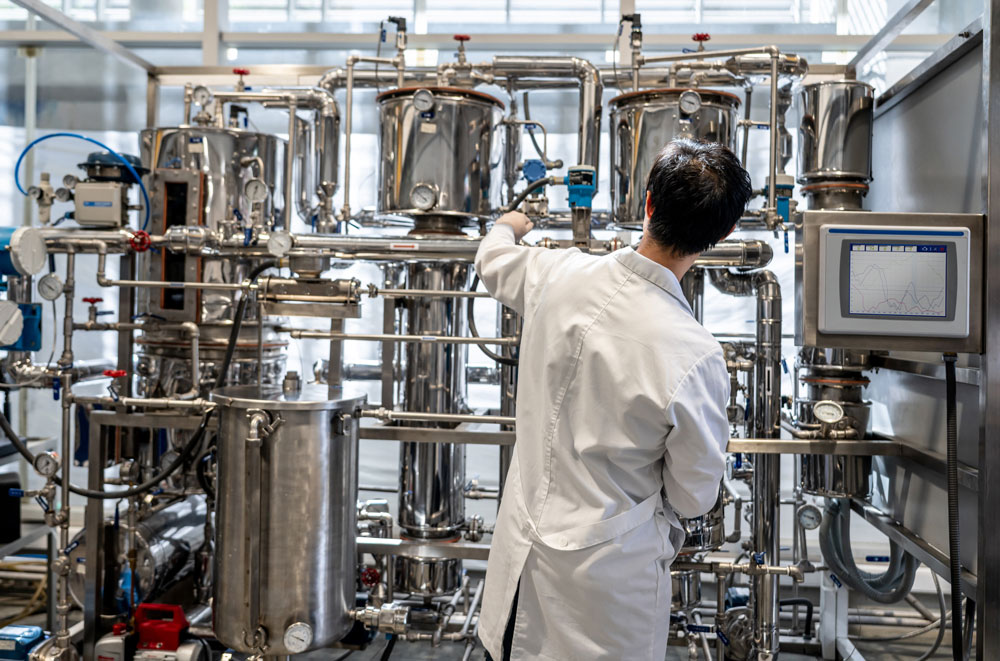Blog

# How do you measure the development of rouge?

October 26, 2021

Rouge is a form of corrosion. Corrosion can be measured by using the Stern-Geary equation first issued in 1957.

Rouge is a form of corrosion. Corrosion can be measured by using the Stern-Geary equation first issued in 1957.

This equation is used to show the corrosion, for each measurement duration time, by measuring the Rp identified as the Resistance Potential.  This equation is based on the imeas  which measures the differential between two electrodes (see picture below), one an anode and the other a cathode, immersed in the water. This highly precise measurement has an accuracy and limit of detection of 1 angstrom (Å). This scientific approach allows for accurate measurements across a very wide range of resistivity values.

As the water interacts with the electrodes a very tiny aspect of corrosion occurs causing the liberation of ferric (Fe) and other metallic ions from the substrate of the metal. Corrosion is the combination of hydroxyl radicals with metallic ions formulating metal oxides. These metal oxides are a direct effect of the corrosion process.  The electrode from where the metallic ions were liberated and combined with the hydroxyl radicals to formulate metallic oxides in the water, is measured for the resistance changes due to the surface of the metal losing ionic composition. This resistance differential is calculated into a nanometer value to show the amount of corrosion since the last measurement was taken.

< Back to the Blog

## More From Our Blog

#### Derouging and Passivation: Economics of the current process

Learn the importance of making data driven decisions for Derouging and Passivation.